We think you are located in United States. Is this correct?

# 8.2 Distance between two points

## 8.2 Distance between two points (EMA69)

A point is a simple geometric object having location as its only property.

Point

A point is an ordered pair of numbers written as $$\left(x;y\right)$$.

Distance

Distance is a measure of the length between two points.

## Distance between two points

Points $$P\left(2;1\right)$$, $$Q\left(-2;-2\right)$$ and $$R\left(2;-2\right)$$ are given.

• Can we assume that $$\hat{R} = 90°$$? If so, why?

• Apply the theorem of Pythagoras in $$\triangle PQR$$ to find the length of $$PQ$$.

To derive a general formula for the distance between two points $$A\left({x}_{1};{y}_{1}\right)$$ and $$B\left({x}_{2};{y}_{2}\right)$$ we use the theorem of Pythagoras.

\begin{align*} AB^{2} & = AC^{2} + BC^{2} \\ \therefore AB & = \sqrt{AC^{2} + BC^{2}} \end{align*}

And:

\begin{align*} AC & = {x}_{2} - {x}_{1} \\ BC & = {y}_{2} - {y}_{1} \end{align*}

Therefore:

\begin{align*} AB & = \sqrt{AC^{2} + BC^{2}} \\ & = \sqrt{{\left({x}_{2} - {x}_{1}\right)}^{2} + {\left({y}_{2} - {y}_{1}\right)}^{2}} \end{align*}

Therefore to calculate the distance between any two points, $$\left({x}_{1};{y}_{1}\right)$$ and $$\left({x}_{2};{y}_{2}\right)$$, we use:

$$\text{distance }\left(d\right) = \sqrt{{\left({x}_{1} - {x}_{2}\right)}^{2} + {\left({y}_{1} - {y}_{2}\right)}^{2}}$$

Note that $${\left({x}_{1} - {x}_{2}\right)}^{2} = {\left({x}_{2} - {x}_{1}\right)}^{2}$$.

The following video gives two examples of working with the distance formula and shows how to determine the distance formula.

Video: 2GBS

## Worked example 1: Using the distance formula

Find the distance between $$S\left(-2;-5\right)$$ and $$Q\left(7;-2\right)$$.

### Draw a sketch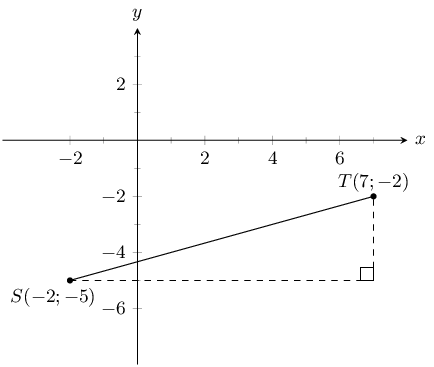### Assign values to $$\left({x}_{1};{y}_{1}\right)$$ and $$\left({x}_{2};{y}_{2}\right)$$

Let the coordinates of $$S$$ be $$\left({x}_{1};{y}_{1}\right)$$ and the coordinates of $$T$$ be $$\left({x}_{2};{y}_{2}\right)$$.

${x}_{1} = -2 \quad {y}_{1} = -5 \quad {x}_{2} = 7 \quad {y}_{2} = -2$

### Write down the distance formula

$d = \sqrt{{\left({x}_{1} - {x}_{2}\right)}^{2} + {\left({y}_{1} - {y}_{2}\right)}^{2}}$

### Substitute values

\begin{align*} {d}_{ST} & = \sqrt{{\left(-2 - 7\right)}^{2} + {\left(-5 - \left(-2\right)\right)}^{2}} \\ & = \sqrt{{\left(-9\right)}^{2} + {\left(-3\right)}^{2}} \\ & = \sqrt{81 + 9} \\ & = \sqrt{90} \\ & = \text{9,5} \end{align*}

The distance between $$S$$ and $$T$$ is $$\text{9,5}$$ units.

temp text

## Worked example 2: Using the distance formula

Given $$RS = 13$$, $$R\left(3;9\right)$$ and $$S\left(8;y\right)$$. Find $$y$$.

### Draw a sketch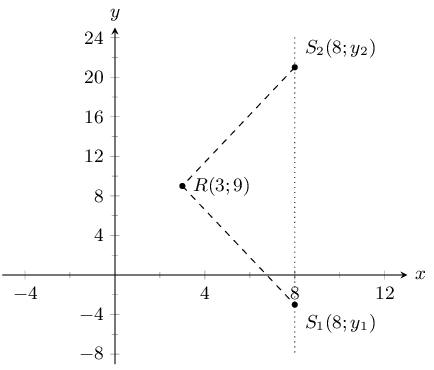Note that we expect two possible values for $$y$$. This is because the distance formula includes the term $$(y_{1} - y_{2})^{2}$$ which results in a quadratic equation when we substitute in the $$y$$ coordinates.

### Assign values to $$\left({x}_{1};{y}_{1}\right)$$ and $$\left({x}_{2};{y}_{2}\right)$$

Let the coordinates of $$R$$ be $$\left({x}_{1};{y}_{1}\right)$$ and the coordinates of $$S$$ be $$\left({x}_{2};{y}_{2}\right)$$.

${x}_{1} = 3 \quad {y}_{1} = 9 \quad {x}_{2} = 8 \quad {y}_{2} = y$

### Write down the distance formula

$d = \sqrt{{\left({x}_{1} - {x}_{2}\right)}^{2} + {\left({y}_{1} - {y}_{2}\right)}^{2}}$

### Substitute values and solve for $$y$$

\begin{align*} 13 & = \sqrt{{\left(3 - 8\right)}^{2} + {\left(9 - y\right)}^{2}} \\ {13}^{2} & = {\left(-5\right)}^{2} + \left(81 - 18y + {y}^{2}\right) \\ 0 & = {y}^{2} - 18y - 63 \\ & = \left(y + 3\right)\left(y - 21\right) \\ \therefore y = -3& \text{ or } y = 21 \end{align*}

### Check both values for $$y$$

Check $$y = -3$$:

\begin{align*} d & = \sqrt{{\left({x}_{1} - {x}_{2}\right)}^{2} + {\left({y}_{1} - {y}_{2}\right)}^{2}} \\ & = \sqrt{{\left(3 - 8\right)}^{2} + {\left(9 + 3\right)}^{2}} \\ & = \sqrt{25 + 144} \\ & = \sqrt{169} \\ & = 13 \end{align*}

Check $$y = 21$$:

\begin{align*} d & = \sqrt{{\left({x}_{1} - {x}_{2}\right)}^{2} + {\left({y}_{1} - {y}_{2}\right)}^{2}} \\ & = \sqrt{{\left(3 - 8\right)}^{2} + {\left(9 - 21\right)}^{2}} \\ & = \sqrt{25 + 144} \\ & = \sqrt{169} \\ & = 13 \end{align*}

$$S$$ is $$\left(8;-3\right)$$ or $$\left(8;21\right)$$.

Therefore $$y = -3$$ or $$y = 21$$.

temp text

Drawing a sketch helps with your calculation and makes it easier to check if your answer is correct.

Textbook Exercise 8.2

You are given the following diagram: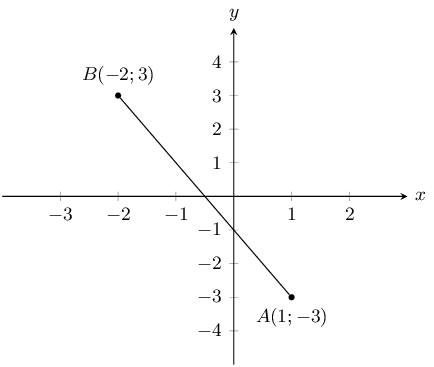Calculate the length of line $$AB$$, correct to 2 decimal places.

First we recall the equation for distance:

\begin{aligned} d_{AB} & = \sqrt{(x_B - x_A)^2 + (y_B - y_A)^2}\\ & = \sqrt{(\text{1} - (-\text{2}))^2 + (-\text{3} - (\text{3}))^2} \\ & = \sqrt{(\text{1} \text{+2})^2 + (-\text{3} -\text{3})^2} \\ & = \sqrt{(\text{3})^2 + (-\text{6})^2} \\ & = \sqrt{\text{9} + \text{36}} \\ & = \sqrt{\text{45}} \\ & \approx \text{6,71} \end{aligned}

You are given the following diagram: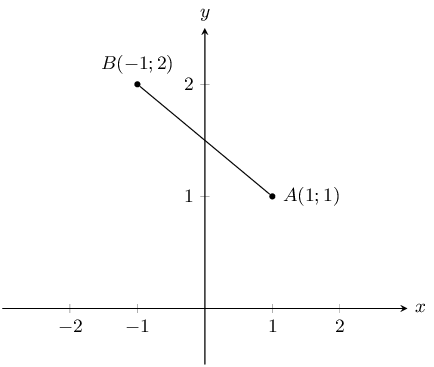Calculate the length of line $$AB$$, correct to 2 decimal places.

First we recall the equation for distance:

\begin{aligned} d_{AB} & = \sqrt{(x_B - x_A)^2 + (y_B - y_A)^2}\\ & = \sqrt{(\text{1} - (-\text{1}))^2 + (\text{1} - (\text{2}))^2} \\ & = \sqrt{(\text{1} \text{+1})^2 + (\text{1} -\text{2})^2} \\ & = \sqrt{(\text{2})^2 + (-\text{1})^2} \\ & = \sqrt{\text{4} + \text{1}} \\ & = \sqrt{\text{5}} \\ & \approx \text{2,24} \end{aligned}

The following picture shows two points on the Cartesian plane, $$A$$ and $$B$$.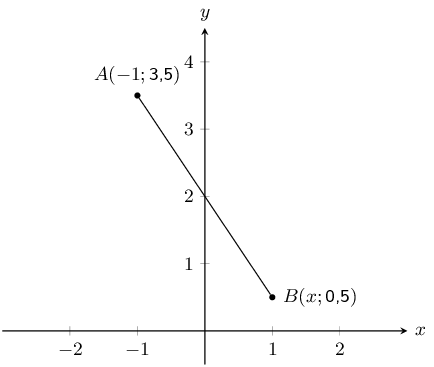The distance between the points is $$\text{3,6056}$$. Calculate the missing coordinate of point $$B$$.

First we recall the equation for distance:

\begin{aligned} d_{AB} & = \sqrt{(x_B - x_A)^2 + (y_B - y_A)^2}\\ \text{3,6056} & = \sqrt{(x - (-\text{1}))^2 + (\text{0,5} - (\text{3,5}))^2} \\ \text{3,6056} & = \sqrt{(x \text{+1})^2 + (\text{0,5} -\text{3,5})^2} \end{aligned}

Now we re-arrange, and solve for the value of $$x$$:

\begin{aligned} (\text{3,6056})^2 & = (x + \text{1})^2 + (\text{0,5} - \text{3,5})^2 \\ \text{13} & = (x + \text{1})^2 + (\text{0,5} -\text{3,5})^2 \\ \text{13} & = (x + \text{1})^2 + \text{9} \\ (x+1)^2 & = \text{4} \\ x + \text{1} & = \pm \sqrt{\text{4}} \\ x & = \pm \text{2} -\text{1} \\ x & = \text{1} \text{ or } -\text{3} \end{aligned}

We now have a choice between 2 values for $$x$$. From the diagram we can see that the appropriate value for this question is $$x = \text{1}$$.

Note that in this case we can use the diagram to check that our answer is valid but we can also calculate the distance of line $$AB$$ using our answer.

The following picture shows two points on the Cartesian plane, $$A$$ and $$B$$.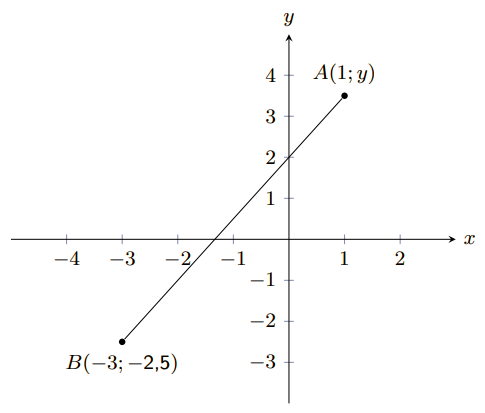The line $$AB$$ has a length of $$\text{7,2111}$$. Calculate the missing coordinate of point $$B$$. Round your answer to one decimal place.

First we recall the equation for distance:

\begin{aligned} d_{AB} & = \sqrt{(x_B - x_A)^2 + (y_B - y_A)^2}\\ \text{7,2111} & = \sqrt{(\text{1} - (-\text{3}))^2 + (y - (-\text{2,5}))^2} \\ \text{7,2111} & = \sqrt{(\text{1} + \text{3})^2 + (y + \text{2,5})^2} \end{aligned}

Now we re-arrange, and solve for the value of $$y$$:

\begin{aligned} (\text{7,2111})^2 & = (\text{1} + \text{3})^2 + (y + \text{2,5})^2 \\ \text{52} & = (\text{1} + \text{3})^2 + (y + \text{2,5})^2 \\ \text{52} & = (y + \text{2,5})^2 + \text{16} \\ (y + \text{2,5})^2 & = \text{36} \\ y + \text{2,5} & = \pm \sqrt{\text{36}} \\ y & = \pm \text{6} - \text{2,5} \\ y & = \text{3,5} \text{ or } -\text{8,5} \end{aligned}

We now have a choice between 2 values for $$y$$. From the diagram we can see that the appropriate value for this question is $$y = \text{3,5}$$.

Note that in this case we can use the diagram to check that our answer is valid but we can also calculate the distance of line $$AB$$ using our answer.

Find the length of $$AB$$ for each of the following. Leave your answer in surd form.

$$A(2;7)$$ and $$B(-3;5)$$

\begin{align*} d_{AB} &= \sqrt{(x_1 - x_2)^2 + (y_1 - y_2)^2}\\ & = \sqrt{(2 - (-3))^2 + (7 - 5)^2}\\ &= \sqrt{(5)^2 + (2)^2}\\ & = \sqrt{29} \end{align*}

$$A(-3;5)$$ and $$B(-9;1)$$

\begin{align*} d_{AB} &= \sqrt{(x_1 - x_2)^2 + (y_1 - y_2)^2}\\ & = \sqrt{(-3 - (-9))^2 + (5 - 1)^2}\\ & = \sqrt{(6)^2 + (4)^2}\\ & = \sqrt{52} \end{align*}

$$A(x;y)$$ and $$B(x + 4; y - 1)$$

\begin{align*} d_{AB} &= \sqrt{(x_1 - x_2)^2 + (y_1 - y_2)^2}\\ & = \sqrt{(x - (x + 4))^2 + (y - (y - 1))^2}\\ & = \sqrt{(x - x - 4)^2 + (y - y + 1)^2}\\ & = \sqrt{(-4)^2 + (1)^2}\\ & = \sqrt{17} \end{align*}

The length of $$CD = 5$$. Find the missing coordinate if:

$$C(6;-2)$$ and $$D(x;2)$$.

\begin{align*} d_{CD} & = \sqrt{(x_1 - x_2)^2 + (y_1 - y_2)^2} \\ 5 & = \sqrt{(6 - x)^2 + (-2 - 2)^2} \\ 5^2 & = 36 - 12x + x^2 + 16\\ 0 & = x^2 - 12x + 36 - 25 + 16\\ & = x^2 - 12x + 27 \\ & = (x - 3)(x - 9) \end{align*}

Therefore $$x = 3$$ or $$x = 9$$.

Check solution for $$x = 3$$:

\begin{align*} d_{CD} & = \sqrt{(x_1 - x_2)^2 + (y_1 - y_2)^2} \\ & = \sqrt{(6 - 3)^2 + (-2 - 2)^2} \\ & = \sqrt{(3)^2 + (-4)^2} \\ & = \sqrt{25} \\ & = 5 \end{align*}

Solution is valid.

Check solution for $$x = 9$$:

\begin{align*} d_{CD} & = \sqrt{(x_1 - x_2)^2 + (y_1 - y_2)^2} \\ & = \sqrt{(6 - 9)^2 + (-2 - 2)^2} \\ & = \sqrt{(-3)^2 + (-4)^2} \\ & = \sqrt{25} \\ & = 5 \end{align*}

Solution is valid.

$$C(4;y)$$ and $$D(1;-1)$$.

\begin{align*} d_{CD} &= \sqrt{(x_1 - x_2)^2 + (y_1 - y_2)^2}\\ 5 &= \sqrt{(4 - 1)^2 + (y + 1)^2}\\ 5^2 &= 9+y^2 + 2y + 1\\ 0 &= y^2 + 2y + 1 + 9 - 25\\ &= y^2 + 2y - 15\\ &= (y - 3)(y + 5) \end{align*}

Therefore $$y = 3$$ or $$y = -5$$.

Check solution for $$y = 3$$:

\begin{align*} d_{CD} &= \sqrt{(x_1 - x_2)^2 + (y_1 - y_2)^2}\\ &= \sqrt{(4 - 1)^2 + (3 + 1)^2}\\ &= \sqrt{3^2 + 4^2} \\ &= \sqrt{25} \\ &= 5 \end{align*}

Solution is valid.

Check solution for $$y = -5$$:

\begin{align*} d_{CD} & = \sqrt{(x_1 - x_2)^2 + (y_1 - y_2)^2}\\ & = \sqrt{(4 - 1)^2 + (-5 + 1)^2}\\ & = \sqrt{(3)^2 + (-4)^2}\\ & = \sqrt{25}\\ & = 5 \end{align*}

Solution is valid.

If the distance between $$C(0;-3)$$ and $$F(8;p)$$ is 10 units, find the possible values of $$p$$.

\begin{align*} 10 & = \sqrt{(8 - 0)^{2} + (p + 3)^{2}} \\ & = \sqrt{8^{2} + (p + 3)^{2}} \\ 100 & = 8^{2} + (p + 3)^{2} \\ 36 & = p^{2} + 6p + 9 \\ 0 & = p^{2} + 6p - 27 \\ & = (p - 3)(p + 9) \\ \therefore p = 3 & \text{ or } p = -9 \end{align*}

Check $$p = 3$$:

\begin{align*} d & = \sqrt{{\left({x}_{1} - {x}_{2}\right)}^{2} + {\left({y}_{1} - {y}_{2}\right)}^{2}} \\ & = \sqrt{{\left(8 - 0\right)}^{2} + {\left(3 + 3\right)}^{2}} \\ & = \sqrt{64 + 36} \\ & = \sqrt{100} \\ & = 10 \end{align*}

Solution is valid.

Check $$p = -9$$:

\begin{align*} d & = \sqrt{{\left({x}_{1} - {x}_{2}\right)}^{2} + {\left({y}_{1} - {y}_{2}\right)}^{2}} \\ & = \sqrt{{\left(8 - 0\right)}^{2} + {\left(-9 + 3\right)}^{2}} \\ & = \sqrt{64 + 36} \\ & = \sqrt{100} \\ & = 10 \end{align*}

Solution is valid.

Therefore $$p = 3$$ or $$p = -9$$.